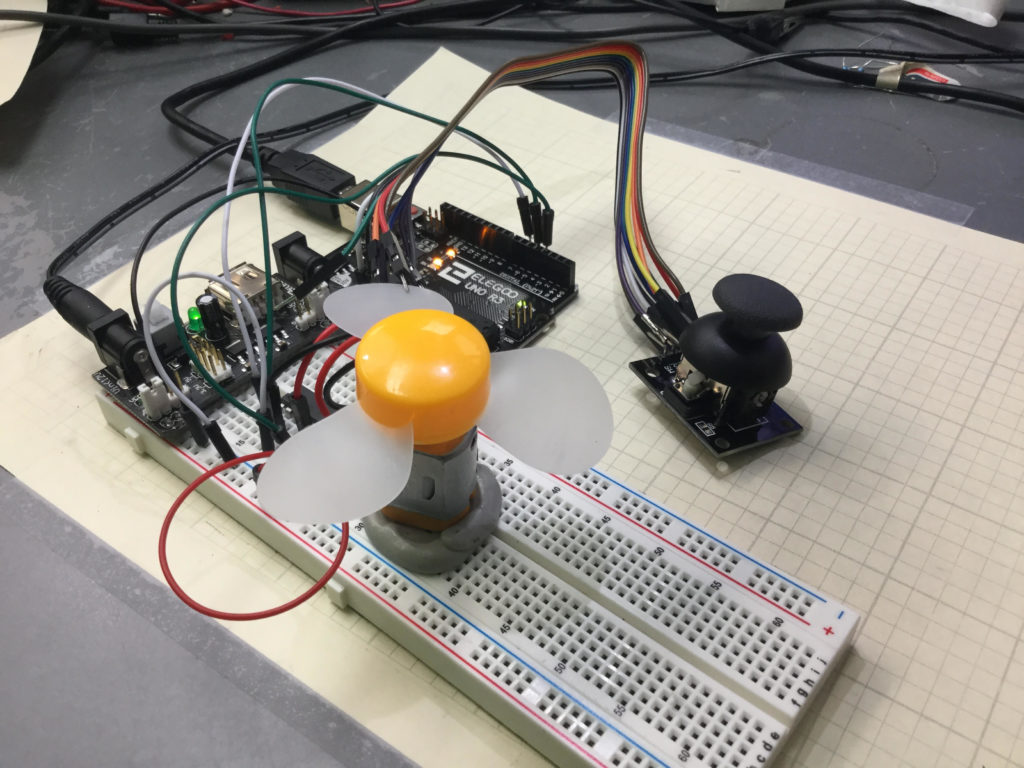# Arduino Tutorial 40: Controlling DC Motor Speed and Direction with Pushbuttons

In this lesson we explore how to control the speed and direction of a DC motor using two buttons. We are using the L293D motor controller and a small DC motor for demonstration purposes. We are using parts from our Elegoo Super Stater Kit, which you can get HERE. The basic circuit was explained in Lesson 37, and we are using that work as a starting point. The schematic below will get you started in connecting your circuit. Be sure and connect one of the Arduino ground pins to the ground rail in the second to the bottom row in the diagram below. It is good practice to connect all your grounds together.

The code we developed in the video lesson is shown below for your convenience.

# Arduino Tutorial 39: Using a Joystick to Control DC Motor Speed and DirectionThis is a simple example of how to control speed and direction of a DC motor with a joystick.

Lesson 37 and 38 showed some preliminary concepts in controlling a DC motor using an arduino and the L293D motor controller. In the video above we show how to control the speed and direction of a simple DC motor using a joystick. In the neutral position, the motor is stationary. Then the speed smoothly increases as you move the joystick forward, until you reach maximum speed.

Lesson 37 and 38 showed some preliminary concepts in controlling a DC motor using an arduino and the L293D motor controller. In the video above we show how to control the speed and direction of a simple DC motor using a joystick. In the neutral position, the motor is stationary. Then the speed smoothly increases as you move the joystick forward, until you reach maximum speed. Similarly, in pulling the joystick back from the neutral position, the motor gradually increases speed in the reverse direction. The diagram below shows the basic motor control schematic we are working from.

In the circuit diagram above, we also add a wire to connect the arduino ground to the power supply ground. It is always good to have all components connected to a common ground rail. Of course, you also need to add the joystick controller as shown in the video.

This is the code we used in this

# Arduino Tutorial 38: Using a Tilt Switch Cut Off With a DC Motor

In this lesson we explore how to add a tilt cutoff to our DC motor project. In many cases, you may want to automatically turn your motors off in the case where your project or robot tips over. The tilt switch is a simple way to do this. The video below takes you through the process step-by-step.

If you need help hooking the circuit up, the diagram below shows you a schematic of what we are working with. All these components are part of our ELEGOO Super Starter Kit.

In addition, you will need to add the tilt switch, as shown in the video.

Below is the code we used, with the tilt switch connected to pin 2 on the arduino.

# Arduino Tutorial 37: Understanding How to Control DC Motors in Projects

In this lesson we learn the ins and outs of controlling a DC Motor from an Arduino. A key aspect of this is to understand you must not connect a motor directly to an arduino. You need to either build a suitable transistor and diode circuit, or you need to utilize a motor controller, such as the L293D motor control chip.